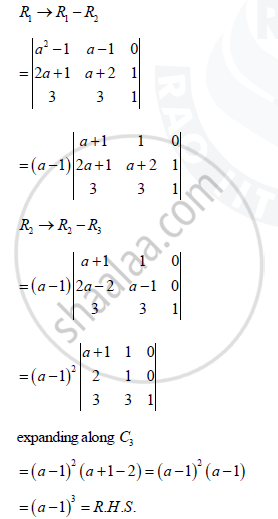Share

# Using Properties of Determinants, Prove That Matrix (Asquare2 + 2a,2a + 1,1,2a+1,A+2, 3, 3, 1 - CBSE (Commerce) Class 12 - Mathematics

#### Question

Using properties of determinants, prove that

|(a^2 + 2a,2a + 1,1),(2a+1,a+2, 1),(3, 3, 1)| = (a - 1)^3

#### Solution

L.H.S = |(a^2 + 2a,2a + 1,1),(2a+1,a+2, 1),(3, 3, 1)|Is there an error in this question or solution?

#### APPEARS IN

Solution Using Properties of Determinants, Prove That Matrix (Asquare2 + 2a,2a + 1,1,2a+1,A+2, 3, 3, 1 Concept: Properties of Determinants.
S